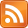# Playing VR Videos in Cardboard Apps

### Matthew Wellings 6-Feb-2016

If you have a VR video (omni‐directional stereo video) that you want to play directly from inside your OpenGL cardboard application or game then you will find it can be played using a simple modification to the code in my earlier post Playing Stereo 3D Video in Cardboard Apps. In that post the video was rendered to a SurfaceTexture using Android's MediaPlayer class to give us video in a usable OpenGL texture. In that post the texture was rendered to a rectangle using different texture co-ordinates for each eye to select the correct image.

VR video is not rendered to a flat surface but to the inside of a sphere with the viewer at its center. The texture co-ordinates across the surface of the sphere corresponds to points in the video according to the equirectangular projection i.e. proportional to the latitude and longitude on the sphere.

Building on the same code as before we just add the code needed to build a construct a sphere with the correct texture co-ordinates to the beginning of the setup() function. The code below is a modification of the esGenSphere() function from "OpenGL ES 2.0 Programming Guide" sample code:

``````short numSlices = 50;
float radius=99.9f; //Radius should be large but keep it between the near and far planes.
short parallel;
short slice;
int numParallels = numSlices / 2;
int numVertices = ( numParallels + 1 ) * ( numSlices + 1 );
int numIndices = numParallels * numSlices * 6;
float angleStep = (2.0f * (float)Math.PI) / ((float) numSlices);

virtualScreenVetrexCoords = new float[3 * numVertices];
videoTextureCoords =new float[4 * numVertices];
videoTextureCoordsTop =new float[4 * numVertices];
videoTextureCoordsBottom =new float[4 * numVertices];
virtualScreenVetrexIndicies = new short[numIndices];

for (parallel = 0; parallel < numParallels + 1; parallel++ )
{
for (slice = 0; slice < numSlices + 1; slice++ )
{
int vertex = ( parallel * (numSlices + 1) + slice ) * 3;
virtualScreenVetrexCoords[vertex + 0] = - radius * (float)Math.sin ( angleStep * (double)parallel ) * (float)Math.sin ( angleStep * (double)slice );
virtualScreenVetrexCoords[vertex + 1] = - radius * (float)Math.cos ( angleStep * (double)parallel );
virtualScreenVetrexCoords[vertex + 2] = radius * (float)Math.sin ( angleStep * (double)parallel ) * (float)Math.cos ( angleStep * (double)slice );
int texIndex = ( parallel * (numSlices + 1) + slice ) * 4;
//Equirectangular:
videoTextureCoords[texIndex + 0] = (float) slice / (float) numSlices;
videoTextureCoords[texIndex + 1] = (float) parallel  / (float) numParallels;
videoTextureCoords[texIndex + 2] = 0f;
videoTextureCoords[texIndex + 3] = 1f;
//Left eye
videoTextureCoordsTop[texIndex + 0]=videoTextureCoords[texIndex + 0];
videoTextureCoordsTop[texIndex + 1]=videoTextureCoords[texIndex + 1]/2f;
videoTextureCoordsTop[texIndex + 2]=videoTextureCoords[texIndex + 2];
videoTextureCoordsTop[texIndex + 3]=videoTextureCoords[texIndex + 3];
//Right eye
videoTextureCoordsBottom[texIndex + 0]=videoTextureCoords[texIndex + 0];
videoTextureCoordsBottom[texIndex + 1]=videoTextureCoords[texIndex + 1]/2f+0.5f;
videoTextureCoordsBottom[texIndex + 2]=videoTextureCoords[texIndex + 2];
videoTextureCoordsBottom[texIndex + 3]=videoTextureCoords[texIndex + 3];
}
}
// Generate the indices
int thisIndex = 0;
for ( parallel = 0; parallel < numParallels ; parallel++ )
{
for ( slice = 0; slice < numSlices; slice++ )
{
virtualScreenVetrexIndicies[thisIndex] = (short)(parallel * ( numSlices + 1 ) + slice); thisIndex++;
virtualScreenVetrexIndicies[thisIndex] = (short)(( parallel + 1 ) * ( numSlices + 1 ) + slice); thisIndex++;
virtualScreenVetrexIndicies[thisIndex] = (short)(( parallel + 1 ) * ( numSlices + 1 ) + ( slice + 1 )); thisIndex++;

virtualScreenVetrexIndicies[thisIndex] = (short)(parallel * ( numSlices + 1 ) + slice); thisIndex++;
virtualScreenVetrexIndicies[thisIndex] = (short)(( parallel + 1 ) * ( numSlices + 1 ) + ( slice + 1 )); thisIndex++;
virtualScreenVetrexIndicies[thisIndex] = (short)(parallel * ( numSlices + 1 ) + ( slice + 1 )); thisIndex++;
}
}``````

Texture co-ordinates generated here are in the range [0, 1] using "slice / numSlices" and "parallel / numParallels". The z and w co-ordanates are 0 and 1 respectively so that we can use the 4x4 matrix from SurfaceTexture.getTransformMatrix(). The texture co-ordinates for the left and right eye are calculated such that they represent corresponding points from the top or bottom image.

It is also important to modify the setup of the view matrix. The old code:

``````Matrix.setIdentityM(videoScreenModelMatrix, 0);
float screenSize=2; //Virtual screen height in meters.
float aspectRatio=16f/9f; //Image will be stretched to this ratio.
Matrix.scaleM(videoScreenModelMatrix, 0, screenSize, screenSize/aspectRatio, 1);
Matrix.translateM(videoScreenModelMatrix, 0, 0.0f, 0.0f, -4.0f);``````

Becomes simply:

``Matrix.setIdentityM(videoScreenModelMatrix, 0);``

Notice that we have removed all references to the aspect ratio. The aspect ratio is irrelevant with this method of rendering as we are guaranteed by the use of a spherical equirectangular projection to always be able to restore the correct image irrespective of the aspect ratio of the video being played. SurfaceTexture always maps the bounds of the video to the texture range [0, 1] providing we transform texture co-ordinates by SurfaceTexture.getTransformMatrix().

Ideally the sphere should be centered at the position of the eye currently being rendered. This will preserve the correct stereo offset. The document Rendering Omni‐directional Stereo Content (Google Inc) states that ODS videos should be rendered such that the "Ray directions are tangent to the circle". This means that objects at infinity are rendered in the same place in both video views. If the sphere is kept in the same place in the scene when rendering each eye, points in the same place in each video view (at infinity in the original scene) will appear on the surface of the sphere. This effect will however not be noticeable if the sphere is sufficiently large compared to the distance between the virtual eyes.

One last alteration is to prevent the drawing of the floor in the scene as it will intrude into the sphere.

### Compatible VR Videos

This code takes the same format as YouTube does. Any video made using the instructions that Google provided for making videos that work with YouTube's VRVideo player should work with this method. This includes videos made using the method described in my earlier post Creating VR video trailers for Cardboard games.